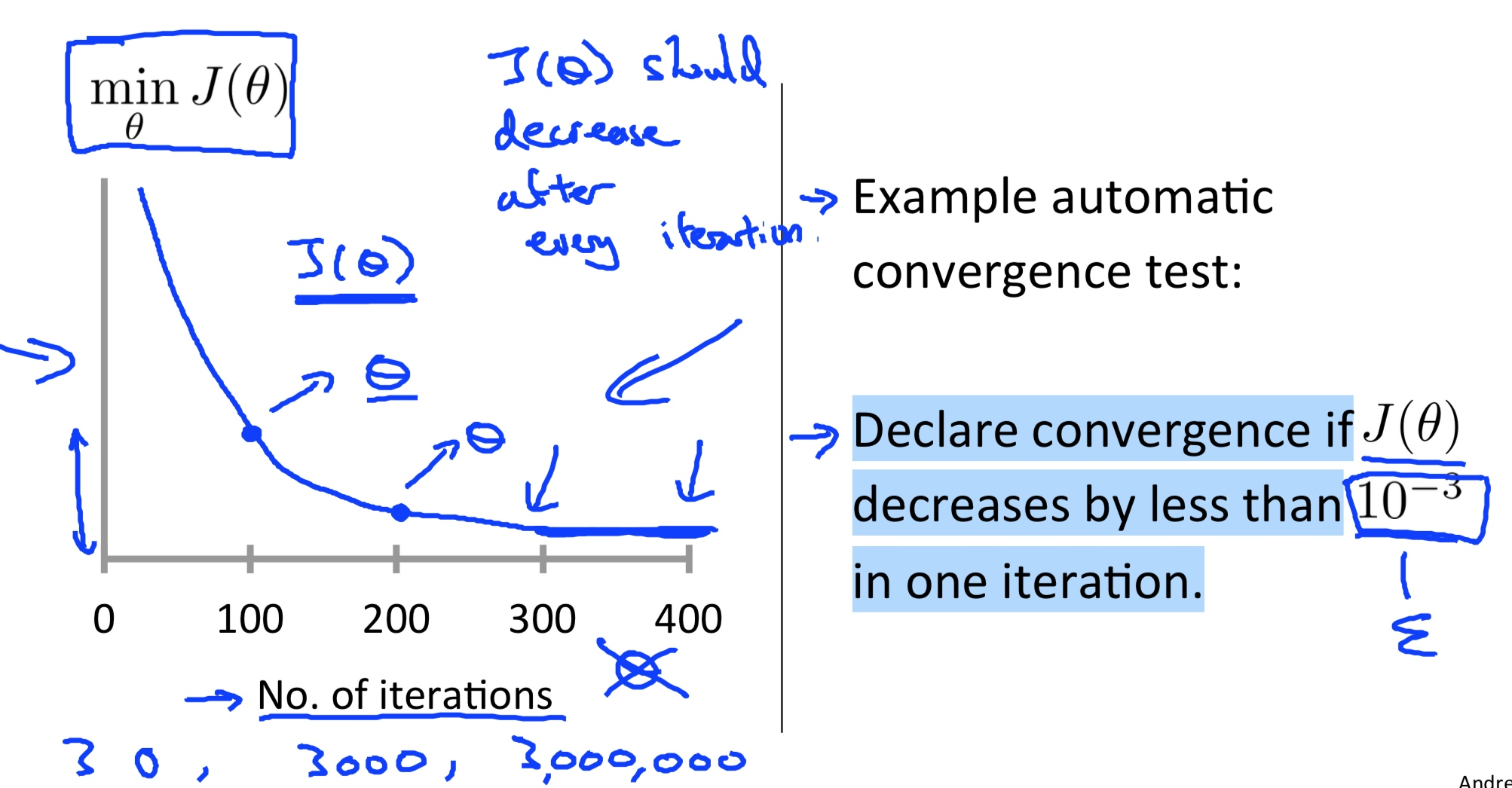# machine learning 笔记

## Linear Regression with one variable

### Notion• m = Number of training examples
• x’s = “input” variable / features
• y’s = “output” variable / “target” variable

### Hypothesis

$h_{\theta}(x) = \theta_0 + \theta_1x$

### Cost function

$J(\theta) = \frac{1}{2m} \sum_{i=1}^m (h_\theta(x^{(i)}) - y^{(i)})^2 = \frac{1}{2m} \sum_{i=1}^m (\theta_0+\theta_1x^{(i)} - y^{(i)})^2$

### Gredient descent

$\theta_j = \theta_j - \alpha \frac{\partial}{\partial\theta_j} J(\Theta) \\ = \theta_j - \alpha \frac{\partial}{\partial\theta_j} (\frac{1}{2m} \sum_{i=1}^m (h_\theta(x^{(i)}) - y^{(i)})^2) \\ = \theta_j - \alpha \frac{1}{m} \sum_{i=1}^m (h_\theta(x^{(i)}) - y^{(i)})(x^{(i)}_j)$

• j = 0

$\theta_0 = \theta_0 - \alpha \frac{1}{m} \sum_{i=1}^m(\theta_0+\theta_1x^{(i)}-y^{(i)})$

• j = 1

$\theta_1 = \theta_1 - \alpha \frac{1}{m} \sum_{i=1}^m(\theta_0+\theta_1x^{(i)}-y^{(i)})x^{(i)}$

(simultaneously update $$\theta_j$$ for all j)

## Linear Regression with multiple variables

### Notion• $$n$$ = number of features
• $$x^{(i)}$$ = input of $$i^{th}$$ training example
• $$x^{(i)}_j$$ = input of $$i^{th}$$ training example 's $$j^{th}$$ feature.
• $$x^{(2)}_3$$ = 2

### Hypothesis derivation

• Previousely: $$h_{\theta}(x) = \theta_0 + \theta_1x$$
• New algorithm: $$h_{\theta}(x) = \theta_0 + \theta_1x_1 + \theta_2x_2 + \theta_3x_3$$

$$X = \begin{bmatrix} x_0\\x_1\\x_2\\\vdots\\x_n \end{bmatrix} \in R^{n+1}$$

$$\Theta = \begin{bmatrix} \theta_0\\\theta_1\\\theta_2\\\vdots\\\theta_n \end{bmatrix} \in R^{n+1}$$

• For convenience of notation, define $$x_0=1$$

$$h_{\theta}(x) = \theta_0x_0 + \theta_1x_1 + \theta_2x_2 + \theta_3x_3 = \Theta^TX$$

### Hypothesis

\begin{align} h_{\theta}(x) &= \Theta^TX \\ &= \theta_0x_0 + \theta_1x_1 + \theta_2x_2+ \cdots + \theta_nx_n \end{align}

### Cost function

\begin{align} J(\theta) &= J(\theta_0, \theta_1, \cdots, \theta_n) \\ &= \frac{1}{2m} \sum_{i=1}^{m}(h_\theta(x^{(i)})-y^{(i)})^2 \end{align}

\begin{align} \theta_j &= \theta_j - \alpha \frac{\partial}{\partial\theta_j} J(\Theta) \\ &= \theta_j - \alpha \frac{\partial}{\partial\theta_j} (\frac{1}{2m} \sum_{i=1}^m (h_\theta(x^{(i)}) - y^{(i)})^2) \\ &= \theta_j - \alpha \frac{1}{m} \sum_{i=1}^m (h_\theta(x^{(i)}) - y^{(i)})(x^{(i)}_j) \end{align}

• j = 0

$\theta_0 = \theta_0 - \alpha \frac{1}{m} \sum_{i=1}^m(\theta_0+\theta_1x^{(i)}-y^{(i)})(x^{(i)}_0)$

• j = 1

$\theta_1 = \theta_1 - \alpha \frac{1}{m} \sum_{i=1}^m(\theta_0+\theta_1x^{(i)}-y^{(i)})x^{(i)}(x^{(i)}_1)$

……

### Feature Scaling

• Make sure features are on a similar scale.
• Get every feature into approximately a $$-1 \leq x_i \leq 1$$ range.

### Mean normalization

Replace $$x_i$$ with $$x_i-\mu_i$$ to make features have approximately zero mean
(Do not apply to ).

E.g.
$x_1 = \frac{size-1000}{2000}$
$x_2 = \frac{bedrooms-2}{5}$

$x_1 = \frac{x_1-\mu_1}{\sigma_1}$
$x_2 = \frac{x_2-\mu_2}{\sigma_2}$

### Learning rate

$\theta_j = \theta_j - \alpha \frac{\partial}{\partial\theta_j} J(\Theta)$

#### “Debugging”: How to make sure gradient descent is working correctly.#### How to choose learning rateSummary:

• If $$\alpha$$ too small: slow convergence
• If $$\alpha$$ too large: $$J(\theta)$$ may not decrease on every iteration; may not converge.

To choose $$\alpha$$, try

$$\cdots, 0.001, 0.003, 0.01, 0.03, 0.1, 0.3, 1, \cdots$$

### Normal Equation

Normal equation: Method to solve for analy2cally.

$\theta = (X^TX)^{-1}X^Ty$

1 Need choose $$\alpha$$ No need to choose $$\alpha$$
2 Need many iterations Do not need to iterate
3 Works well even n is large Need to compute $$(X^TX)^{-1}$$, time complexity O(n3)
4 Slow if n is very large

## Linear Algebra:

### Matrices and vectors

• Matrix:
• Dimension of matrix:
• Matrix Elements
• Vector

• Inverse
• Transponse

## Octave Tutorial

### Vectorial implementation

$\begin{split} &\theta_j = \theta_j - \alpha \frac{\partial}{\partial\theta_j} J(\Theta) \\ &= \theta_j - \alpha \frac{\partial}{\partial\theta_j} (\frac{1}{2m} \sum_{i=1}^m (h_\theta(x^{(i)} - y^{(i)}))^2) \\ &= \theta_j - \alpha \frac{\partial}{\partial\theta_j} (\frac{1}{2m} \sum_{i=1}^m (\theta_0+\theta_1x^{(i)} - y^{(i)})^2) \\ &= \theta_j - \alpha (\frac{1}{m} \sum_{i=1}^m (1+x^{(i)})) \end{split}$